# Data Structures

In the following sections, we will cover some of the standard data structures.

#### Static Arrays

Games rarely need to work with single instances of data. More frequently, you will need to store lists of similar type elements: triangles in a mesh, enemies in a game level, and so forth. Many different structures can be employed to fulfil this task. The simplest of them is the static array, which basically allows the developer to store a list of elements that will not change during the program's life cycle. If you need data to vary (for example, add or remove enemies from a dynamic enemy pool), a more involved solution (such as a linked list) might be better suited. But in many situations (such as the vertices in a static mesh mentioned earlier), you just need to store a linear array of data.

This structure has the following general definition:

` class arrayoftype         {         type *data;         int size;         arrayoftype(int);         ~arrayoftype();         }; `

The constructor would require an initialization such as:

` arrayoftype::arrayoftype(int psize) { size=psize; data=new type[size]; // subsequent code can be used to access and initialize each element } `

The destructor would be as simple as:

` arrayoftype::~arrayoftype() { if (data!=NULL) delete[] data; } `

An added benefit of static types comes in search speed. If the element's position in the array can be considered a primary search key (in database programming terms), you get O(1) access time. And there's more: Imagine that you don't have a primary key as the element position. If you can at least guarantee that elements can be ordered by the value of the primary key (for example, alphabetically or in ascending numeric value), you can still get O(log2 # of elements) searches, which is better than the linear cost you are used to. Remember that we need to incur the lowest possible cost in our routines. Linear cost means cost that is linear to the number of processed elements, whereas logarithmic cost means cost is log(number of elements). Because the logarithm function grows more slowly, we will prefer this type of cost over linear cost.

To achieve this, you need to use a simple but effective algorithm, which is called a binary or dychotomic search depending on the book you read. Here is the core algorithm. For simplicity, I will assume you want to search in a fully ordered passport number list.

` typedef struct       {       int passportid       char *name;       } person; class people       {       person *data;       int size;       person *seek(int);       }; `

The seek method returns a pointer to the person if found, or NULL if the person does not exist. Here is the code:

` person *people::seek(int passid) { int top=size-1; int bottom=0; // this loop stops when we have narrowed the search to just one element while (top-bottom>1)    {    // compute the midpoint    int mid=(top+bottom)/2;    // check which sub-range we want to scan further    if (passid>=data[mid].passportid) bottom=mid;    else top=mid;    } if (data[bottom].passportid==passid) return (&data[bottom]); else return NULL; } `

Clearly, using static arrays makes sense in many scenarios. Their maintenance is really straightforward, and access functions are efficient. However, sometimes we will need dynamic structures that can insert and delete elements at runtime. Then we will need to revert to some more powerful tools.

A linked list is an extension of the static array. Like the static array, it is designed to store sequences of equal-typed elements. But linked lists have an added benefit: The sequence can grow or shrink dynamically, accommodating a variable number of elements during the structure's life cycle. This is achieved by using dynamic memory for each individual element and chaining each element to the next with pointers. The basic implementation of a linked list follows:

` typedef struct       {       // here the data for the element       elem *next;       } elem; class linkedlist       {       elem *first;       elem *current;       }; `

Notice how we keep two pointers, one to the first element in the list (which we will not move) and another to the current element, which we will use for searches and scans in the list. Clearly, there is no way of performing a random access to a given position unless you loop through the list until you reach the target element. Thus, the linked list is especially well suited for sequential traversals, but not so much for random access.

Linked lists are superior to arrays because they allow us to resize the data structure as needed. On the other hand, there are two potential issues with this structure. First, it requires some care with memory allocation to make sure leaks do not occur. Second, some access routines (searches and random reads) can be slower than with arrays. In an array we can use direct access to any position. From this direct access, some clever algorithms (such as dychotomic searches) can be implemented on arrays. But in a linked list, we must use pointers to access elements sequentially. Thus, searches are linear and not logarithmic in cost.

Deletions are also a bit more complicated. We would need a pointer to the previous element in order to fix the pointers in the fashion that is depicted in Figure 3.1. This can be solved in search and delete routines by always storing the required pointer to the previous element. This works fine with elements in-between, but requires a specific routine to delete the first element in the list, which does not have a "previous." A different, more popular approach is to use doubly-linked lists.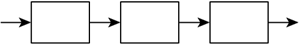A doubly-linked list is a variant of the regular linked list that allows bidirectional scanning by providing, for each element, both a pointer to the next and a pointer to the previous element (see Figure 3.2). This way some routines, such as the deletion routine, are easier to code at a higher memory footprint due to the added pointers. The new data structure could be defined as:

` typedef struct       {       // here the data for the element       elem *next;       elem *prev;       } elem; class linkedlist       {       elem *first;       elem *current;       }; `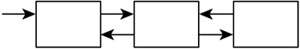Doubly-linked lists offer easier insertion and deletion because we can always access both the previous and next elements to perform pointer reassigning. On the other hand, insertion or deletion at either endpoint is a special case because we can't access the next element (in the last case) or the previous one (in the first case).

But what if we want to store a sense of order so we know when each element was inserted?

#### Queues

A queue is a variant of the linked list. The main difference is that insertions to the queue are always performed at the end of the list, and access to the elements is limited to the element at the beginning (see Figure 3.3). This way a software queue resembles a real-world queue, such as a supermarket waiting line. Customers join the line (hopefully) at the end, and only the customer at the beginning is effectively paying the cashier.

` typedef struct       {       // here the data for the element       elem *next;       } elem; class queue       {       elem *first;       elem *last;       void insertback(elem *);       elem *getfirst(elem *);       }; `
##### Figure 3.3. Queues.Queues are extremely useful for a variety of applications. Generally speaking, queues are used to store lists in chronological order: messages received in a network connection, commands given to a unit in a real time strategy game, and so on. We will insert elements at the end, and extract them in the beginning, thus preserving the order of arrival. This list can be either variable or fixed in size. Fixed-size queues are usually implemented via circular queues and are used to store the N most recent elements. New elements overwrite the oldest ones.

A popular use of circular queues can be found in many 3D games. Imagine that you want to paint the footsteps of your character or bullet marks on the walls. You can't afford to paint lots of these elements because it would clog your rendering engine. So, you decide to paint the last N. Clearly, this is a perfect scenario for circular queues. As the queue gets filled, older elements will be deleted, so only the last footsteps will be painted.

#### Stacks

A stack is another variant of the linked list scheme. This time, though, elements are inserted and popped out at the same end of the structure (see Figure 3.4). This way, a last in, first out (LIFO) behavior is implemented. Stacks thus mimic a pile of paper. The last piece of paper put on top of the list will be the first to be taken out. Here is the code structure for a generic stack:

` typedef struct       {       // here the data for the element       elem *next;       } elem; class stack       {       elem *first;       void push(elem *);       elem *pop();       }; `
##### Figure 3.4. Stack.Stacks are useful in situations where you want to access elements in a geometrically meaningful way: inventories, items dropped to the ground, and so on. Stacks are also used in those cases where newer information makes old information less relevant, as in a cache. The LIFO behavior gives priority to newer elements.

#### Deques

When using both a stack and a queue, data structure is sometimes a waste of code, especially considering that both structures are extremely similar (with the exception of the points of insertion and deletion). A popular alternative to having two separate structures is to have a single object that encapsulates both the queue and the stack. This is called a deque (double-ended queue), and it allows both push and pop operations to be performed at both endpoints (see Figure 3.5). Queues and stacks are so similar internally, that it makes sense to have a single structure that can handle both behaviors.

##### Figure 3.5. Deque.The profile for this new data structure is as follows:

` typedef struct       {       // here the data for the element       elem *next;       elem *prev;       } elem; class deque       {       elem *first;       elem *last;       void push_front(elem *);       elem *pop_front();       void push_back(elem *);       elem *pop_back();       }; `

Notice how we have chosen to implement the deque as a doubly-linked list. Although this is the most popular alternative (remember that we need to delete elements from both sides), some clever coding techniques allow deques to be implemented as simple linked lists, with a significant saving in pointers. It is also interesting to remember that being both a first in, first out (FIFO) and LIFO structure, we do not provide any member operation to scan the list or access elements other than the extreme ones. We will never perform random accesses. On the other hand, it is useful to have an extra integer attribute that holds the number of elements currently being held by the deque so we have a size estimate. This is a popular query, which can be implemented easily by initializing the counter to zero at creation time and incrementing its value in each push and decrementing it on each pop.

#### Tables

Tables are sequential structures that associate data elements with an identifying key. For example, you could have a table where you store people's names, identified by their passport number.

Tables can have many registers (sometimes referred to as rows) and also many fields per register (also referred to as columns). Their main use is in databases, although data-rich games frequently use tables to store anything from weapon types and their characteristics to enemies.

Tables exist in many forms. In the simplest case, a static array can be considered a table where the position of the element acts as the key. In this case, the table is single key (there is only one field that can act as the key), and the key is nonrepetitive (a position can only appear once) and exhaustive (all key values are assigned). This is the optimal case because access time is O(1), and it can be used in some situations. For example, imagine that you are building a role-playing game and need to store weapons and their stats. You could create a table with several columns (for the weight, damage, size, and so on) and use the first one (which will end up as the weapon position in the array) as an identifier. Thus, the long sword could be weapon number 0, the bow number 1, and so forth. Programmers must try to implement tables in such a primitive way when possible to maintain optimal performance.

Unfortunately, most tables are more complex. To begin with, it is hard to find exhaustive keys. For example, you can have a list of customers with their passport numbers, but it is unlikely that all passport identifiers will be occupied. We must divide this problem into two separate contexts, each with a different solution. Will the data be mainly static, and thus insertions and deletions will be nonexistent (or very seldom), or is the table going to involve frequent structural changes?

In static cases, sorting by key and storing data in ascending order in a static array can be a good choice. You can still access data at O(log2 number of elements) by using binary searches. If you need to perform an insertion, you will definitely need to resize the array and make sure the new element keeps the array sorted. Deletions also need array resizing, but overall, both operations can be performed in O(n) cost. Although these operations are rare, the structure is still quite efficient.

##### Hash Tables

A more involved structure can handle both static and dynamic data as well as nonexhaustive keys. Its downside is more memory overhead and slightly more complex coding. The structure is called a hash table, and its main feature offers nearly constant access time for element search, insertion, and deletion.

A hash table (depicted in Figure 3.6) consists of a data repository that effectively holds the table data, coupled with a function that transforms input keys into pointers to data in the repository. The hashing function "scatters" keys uniformly in the repository space (hence the name), making sure available space is occupied in a homogeneous way. Hashing functions are not magic, however. They provide us with a shortcut to data, but sometimes we will need a bit of processing to reach the element we are looking for. Let's take a look at an example based again on a list of people identified by passport number. We will begin by allocating the following structure:

` class hash_table       {       dlinkedlist data;       void create();       elem *seek(int key);       void insert(elem *el);       void delete(int key);       dlinkedlist *hash_function(int key);       }; `
##### Figure 3.6. Hash table.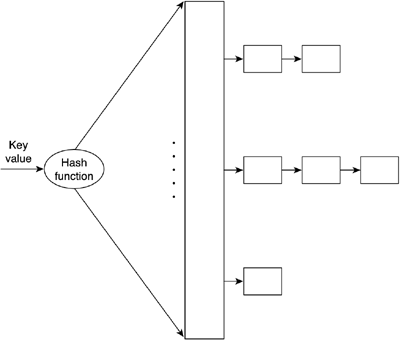In this example, our hash table will be implemented as an array of doubly-linked lists. The hash function then converts any input key into a pointer at the beginning of one of the lists, where elements are really stored. Here is the source code for the hash function:

` dlinkedlist *hash_table::hash_function(int key) { int pos=(key%100) return &data[pos] } `

Notice how the hash function simply selects the last two digits of the password and uses them to select the linked list where data will be stored. This choice is not, however, trivial. Programmers must select hash functions that scatter data as uniformly as possible, or access time will degrade in those table positions holding more data than others. I have thus assumed that the last two digits in a passport are basically randomly distributed, so using them as a hash function makes sense, whereas selecting the first two digits would probably yield a poorer distribution pattern.

Notice also how hash functions do not really provide direct shortcuts to the element we are looking for. They only point us in the right direction because there might be more than one element in the list. We need to perform a linear search in this (hopefully small) list to find the element we are looking for. Selecting the right array size and hash function is essential to guarantee nearly O(1) access time. If we fail to do so, the table will become saturated, and performance will drop rapidly.

Hash tables are quite popular in mainstream applications that need to perform searches in large data banks. Although memory hungry, they can cut down access times dramatically. For example, imagine that you need to hold about 10 million registers of people by their passport number for a government application. By extending the previous ideas, we could use a larger array (say, 10,000 entries long) and hash with passport modulo 10,000. The average list length would end up being approximately 1,000 units. Now, compare scanning a list of 1,000 units with lists of 10 million. Additionally, you can increase or decrease search speed at will just by scaling up the data structure. Obviously, there is a downside to all this. You must be ready to purchase lots of memory because a larger array of doubly-linked lists clearly needs lots of pointers. Assuming you use 32-bit pointers, a 10,000-unit long array with each entry storing a 1,000 element doubly-linked list occupies for each list:

1,000 elements consisting of two pointers each, plus a variable amount of data (say, 100 bytes): totals 108,000 bytes per list

Then, the whole structure needs 10,000 lists and 10,000 pointers to the first element. The grand total is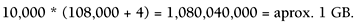Now, a static array holding the entire database, but without the hash table overhead, would occupy 10 millionx100 bytes, for a grand total of 1 billion bytes. If you compare both quantities, you can get an approximation of the hash table overhead: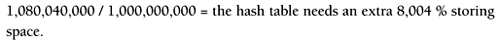Clearly, data structures holding large records will have less overhead because the size of the pointers will slowly become negligible. On the other hand, lightweight structures are not very suitable for hash tables (in terms of memory footprint, not in terms of speed) because the list pointers can significantly add to the memory use.

##### Multi-Key Tables

Let's imagine for a second that we need to create a table that we will access by either of two different values efficiently. For example, we might have a table of people that we want to access by passport number or by name (I will assume no two people have the same name).

We could decide which of the two keys is more important, and then prioritize that key, making it a hash table or an ordered list. But what if we really need to access the table using both keys? The best option here is to use two hash tables (one per key) connected to doubly-linked lists that can be traversed horizontally (for the first key) or vertically (for the second). Take a look at Figure 3.7 to better understand the notion of vertical and horizontal in the preceding example.

##### Figure 3.7. Multi-key table.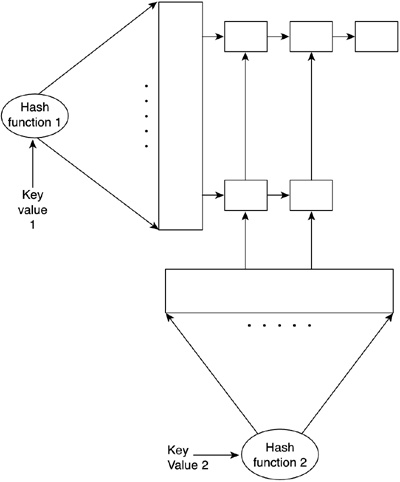This way both keys can benefit from the speed gain found in hashing techniques. Note, however, that this choice is not for the faint of heart. Coding the double hash table can be a complex task because each element is part of two lists, and insertions and deletions get a bit more complex. Also, remember that you will be incurring additional memory overhead for the second table because you will need extra pointers and an extra array to handle the new key.

#### Trees

A tree is a data structure consisting of a series of nodes. Each node holds some relevant information as well as pointers to other nodes in the tree. What makes trees different is the way these node pointers are laid out. Starting from a root node, each node has pointers to nodes that are "descendants" of it. The only restriction is that a given node must only have one direct father (except for the root node, which has none). Thus, a treelike structure appears.

Trees are often approached using a nature-like metaphor: root, branches, leaves, and so on. But this is seldom useful when trying to explain what trees can be used for. It is better to think of a tree as a road that divides itself all the time, where each final destination can only be reached through a single path (due to the single parent condition) no loops, no confusing crossings. Just think of it as an ever-branching road. Clearly, you will discover that trees are great tools to classify large amounts of information. If you are able to find a classification key, it's all a matter of using the road "divisions" (each node) to ensure that you reach your destination quickly the faster the road branches (thus, the more descendants for each node), the faster the access. Let's now examine different types of trees and their uses.

##### Tree Types and Uses

Tree types begin with simple binary trees and run the gamut from N-ary trees to tries.

##### Binary Trees

A binary tree is a data structure where each node has exactly two descendants. This is usually achieved with two pointers called the left and right descendant. Leaf nodes (nodes that do not have descendants) still have two pointers, but they are initialized to NULL values to indicate that no further descendants exist. Data in trees is stored in each node, although some types of trees restrict this in some ways data is held only at leaves, only at nonleaves, and so on.

The general data structure is

` typedef struct       {       tree *left;       tree *right;       // here goes the real data from the node       (...)       } tree; `

Binary trees are widely used in a variety of contexts. Their branching nature makes them ideal as classification devices with logarithmic search time. Each branching divides the search space by a factor of two, granting speedy searches. All we need to do is classify information stored in the tree according to whichever criteria we might need: numeric, spatial, and so on.

As an example, we can implement a Binary Search Tree (BST), which is a tree data structure that is used to speed up element searches. A BST is just a regular binary tree with the following added rule:

For each node in the tree, the key value of all elements in the left subnode must be smaller than the key value of all elements in the right subnode.

This way we can scan the tree quickly, as in a binary search. The added benefit is that the tree is dynamic in nature, so we get O(log2n) access time with dynamic size.

But BSTs can degrade when the tree is unbalanced. Some leaves are much farther from the root than others, so not all branches have the same height.

As shown in Figure 3.8, an unbalanced tree can spoil the access time to make it linear, even if it complies with the BST definition.

##### Figure 3.8. AVL tree.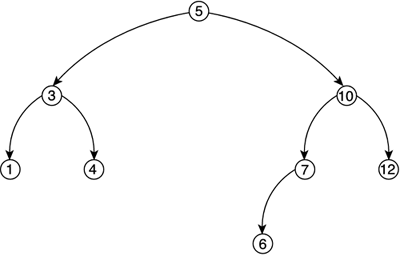So, a new type of binary tree must be introduced. This tree is called an AVL-tree (AVL are the initials of the discoverers of the data structure). An AVL tree is a BST with the additional restriction that for every node in the tree, the depth (in levels) of the left subtree must differ at most by one unit from the depth of the right subtree. Take a look at Figure 3.8 for an example. AVL trees keep the tree balanced, ensuring optimal access time. But efficiency comes at a price. Insertions and deletions into the tree must be done carefully, rebalancing the tree in the process to ensure that the new tree is still an AVL. This rebalancing is achieved by a recursive series of branch shuffles.

We have seen how a binary tree can be a very good structure to use for classifying items. But that classification does not necessarily need to be based on a scalar or alphanumeric value. A binary tree can be best described as a spatial sorter. Once fed with spatially relevant data such as level geometry, it can effectively classify it, sort it, and so on, according to different criteria: which triangles are closest to the player, which convex cells are there, and so on.

We will call this specific tree a Binary Space Partition (BSP) tree. BSPs are very popular in game development. For example, the Quake engine is built using them, as are all of id Software's titles since Doom.

But many other games use them frequently because they provide a very good spatial sorting behavior. Here the idea is to use each node to recursively split the level geometry in half by using a splitting plane. If the planes are chosen properly, the resulting data structure will help us ask questions such as which triangle is closest/farthest, how many convex areas are there in this geometry, and so on. BSP trees will be covered in detail in Chapter 13, "Indoors Rendering."

##### N-ary Trees

Binary trees are used to classify data. But sometimes more branches will lead to better classification power. Here is where N-ary trees can be useful. Complex problems are hard to model, even with a binary tree. N-ary trees can use fixed or variable branching factors. In a fixed branching tree, every node in the tree has the same number of descendants. Quadtrees and octrees have branching factors of 4 and 8 as you will soon see. These trees are easier to code at the cost of reduced flexibility. In a variable branching tree, such as a trie, each node can have a different number of descendants. This is harder to code because we need descendant lists instead of fixed pointers, but it provides an additional degree of flexibility in some cases. A trie used as a dictionary can have a branching factor between 1 and 26 depending on the node.

Quadtrees and octrees are the natural extension of BSP trees. They are fixed-branching, four- and eight-connected trees, respectively. A quadtree is useful in 2D scenarios, whereas an octree is designed to work on 3D data sets. Quadtrees are used in many areas of game programming. In the next chapter, we will see how they can help us perform fast spatial queries, whereas Chapter 14, "Outdoors Algorithms," deals with uses of quadtrees for terrain rendering.

The construction scheme for both is identical, but I will focus on a quadtree now for the sake of simplicity.

A quadtree is built by dividing the incoming 2D data set into four quadrants by using two centered, axis-aligned cutting planes. All four quadrants are thus identical in size. Geometry that crosses quadrant boundaries can either be divided or stored in the quadrant where its major part exists, thus creating different quadtree variants. What should be noted, though, is that not all four quadrants will contain the same amount of data only in a perfectly homogeneous scenario would this be the case. Thus, quadtrees apply the subdivision method recursively, building tree nodes in the process until a threshold is met. Thresholds are usually expressed in the form "each leaf node can contain N elements at most," but can take alternative approaches. You can see a graphic representation of a quadtree along with its resulting tree structure in Figure 3.9.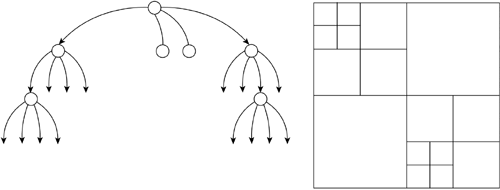Octrees are the 3D counterpart to quadtrees. Instead of dividing each node into four subnodes (usually dubbed front-left, front-right, back-left, back-right), octrees use three axis-aligned and centered planes to create eight octants.

Quadtrees and octrees are powerful tools for visibility computation. They can be used both in indoors and outdoors scenarios to quickly determine visible regions of the game world. So, we will leave more in-depth explanations for Chapters 13 and 14, where we will explore some of their properties and implementation details.

##### Tries

A trie is an N-ary tree where each node can have a variable number of descendants. Each node represents a character or digit in a sequence, so each unique path from the tree root to one leaf node represents a unique key value. It is a very specific data structure whose main use is the fast classification and validation of data, which can be represented as character sequences, ranging from VISA numbers to a word dictionary. For example, a trie that stores the words "cat," "cow," "coalition," "chamber," and "chameleon" can be seen in Figure 3.10.

##### Figure 3.10. Trie.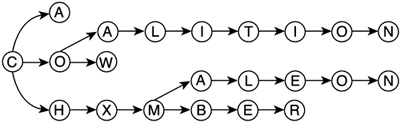Tries offer very fast access time, which is sustained as the structure gets larger and larger. Because the branching factor is very high, we can ensure rapid convergence. For a word N characters long, only N levels are visited; each one branching to between 1 and 26 descendants. Thus, cost ranges from an extremely degenerate case of O(number of letters) for a trie storing only one word to O(log26 number of letters) for a trie that stores all possible letter combinations. For numeric tries, O(log10 number of digits) is the cost of a full trie.

As for insertions and deletions, they are just variants of the core search routine. To delete a sequence, we must search for it; if we reach its end node (meaning the word is effectively in the trie), we must backtrack, deleting all the ascendant nodes until one of them has a descendant other than the one we are deleting. This way we ensure that we do not delete any other sequence as a side effect. You can see this in action in Figure 3.11. Insertions are similar. We begin by searching the sequence. In the process, we will reach a node where no possible advance can be made. We have identified part of our word, but the rest is simply not there. All we have to do is create a descendant subtree that contains the required characters to complete the new sequence. All in all, it seems obvious that both methods come at a worst-case cost of O(number of characters) after the initial search has been completed.

##### Figure 3.11. Deletion in a trie.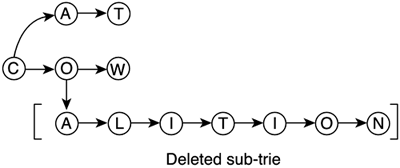##### Tree Traversal Operations

Given the branching nature of trees, traversal cannot be performed in a sequential manner as in a list or table. We need some specific traversal routines that help us access data held by the tree in an intuitive and efficient way. These operations are the addition and subtraction equivalents for trees.

The most usual operation we will perform is the ordered traversal, which is a recursive scan of the whole tree using classification criteria. There are three classical traversal algorithms: pre-order, in-order, and post-order. They differ in the order in which they visit a node and its left and its right descendants.

In pre-order traversals, we first visit the node itself, and afterward we recursively scan the left and right subtrees using pre-order traversal. Graphically speaking, it involves scanning the tree visiting left subtrees prior to right ones and outputting the nodes as we "see" them in the first place.

In-order traversal visits the left subtree, then the node itself, and then the right subtree. It is equivalent to scanning a tree in the usual fashion (first left subtrees, then right) and outputting the nodes only after all the left subtree has been outputted.

The last traversal is the post-order, which is simply visiting the left and right subtrees, and only when these traversals each one recursive in nature have been performed, output the node itself. In practical terms it means visit in the usual way and output the node only if both subtrees have been fully outputted before. Take a look at Figure 3.12 to better understand each traversal method in a real example.

##### Figure 3.12. Pre-order, in-order, and post-order.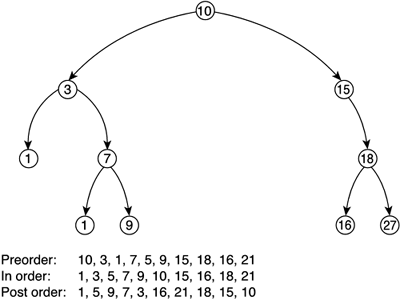#### Priority Queues

A priority queue is an extension of the queue model that incorporates a sense of priority. When newer elements are inserted, they can "pass" in front of those with lesser priority. Thus, if a top priority element is inserted in a very long queue, all the other elements will be displaced by it.

Priority queues are great for representing commands. You can use them to give orders to troops so they prioritize them, and so on.

They are also very useful in sorting algorithms. In fact, one of the fastest algorithms uses a priority queue implementation called a heap to achieve near-optimal O(n*log n) results. The algorithm, dubbed heapsort, basically introduces the elements in the queue one by one, and then extracts them. As each insertion reshuffles the queue using the priority, the elements are extracted in order.

Priority queues can be implemented in a variety of ways. As a first but not very efficient solution, you could choose to implement them as a standard queue (enriching each element with priority information). Then, the insert() routine should be rewritten, so the elements inserted at the back are effectively pushed forward to the position their priority assigns them to. This approach would yield a not so stellar O(n) performance for each insertion.

A better alternative is to use a heap (see Figure 3.13), which is a priority queue implemented as a static array with some clever access routines. To understand heap structures, we must first learn a bit about ordered binary trees. These data structures are defined so that the descendants of each node have inferior value (or priority) than the node itself. The tree is complete, meaning there are no leaves with only one descendant (except for the ones at the end of the tree). Then, the tree is used to sort elements, so high value elements lie closer to the top and, within a specific level, leftmost nodes have higher priority than rightmost nodes. Thus, if a high-priority element is inserted into the tree, a series of level swaps will be performed so the new element is "promoted," and previously inserted elements are pushed to lower levels of the tree. The new element will reach its destination level in a O(log2 number of elements) time, because the logarithm is the measure of the number of levels in the tree. Then, some minor rehashing inside the level may be needed to ensure intralevel ordering is kept as well.

##### Figure 3.13. Heap.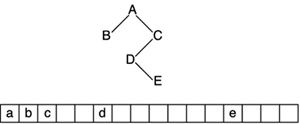A heap is nothing but a binary ordered tree implemented in an array.

The first position of the array is the root, and subsequent positions represent the levels of the tree from root to leaves, and from leftmost to rightmost node. This representation has an advantage: Given an array location (and hence a tree node), we can compute the position of the ascendants and the descendants with a simple formula. If you think about it for a second (and maybe do a couple drawings), you will discover that given a node at position n in the array:

• The ascendant is at position n/2, truncating if needed.

• The left descendant lies at the position 2*n.

• The right descendant lies at the position (2*n)+1.

These equations make access more efficient and ensure that a heap can insert and remove elements quickly. The only downside is that the priority queue that results from the heap structure will always have a fixed length for the static nature of the array. This is rarely a problem, because most situations requiring a priority queue can actually restrict the maximum number of elements to be queued at any given time.

#### Graphs

A graph is a data structure composed of a series of nodes and a series of connections between them. We can navigate through the node list not by using a sequence, but simply by advancing through the connections of each subsequent node. These connections are formally called transitions because they allow us to move from one state to another.

Graphs are a very extensive subject on their own, defining a whole area of knowledge called Discrete Mathematics or Graph Theory. They provide very intuitive ways of representing relational data, such as those in the following list:

• Locations on a map with roads and traveling distances

• State machines with conditions to change from one state to another

• People with relationships between them (friendship, and so on)

• Board game configurations with possible moves

Anything that involves a group of entities and relationships between them can effectively be represented as a graph. But there is more to graphs than representing data: Powerful analysis algorithms have been developed, which scan graphs to calculate valuable results, such as:

• What is the shortest route (and how long will it take) from city A to B?

• What is the chance of a state machine reaching a given state?

• Given two people in a group, do they have any friends in common?

• In a chess game, what is the best move to achieve victory?

Graphs are so broad that many different classification criteria exist. To begin with, graphs can be directed or nondirected. In a directed graph, a transition between two nodes specifies which is the target and which is the destination, so each transition can only be traversed in one of two ways. A transition in a nondirected graph can be traversed in both ways equally. A road map, for example, would generally be nondirected, whereas a street map is often directed because some streets are one-way only. Another classification that is sometimes handy distinguishes between cyclic and acyclic graphs. A cyclic graph allows you to visit the same node twice by using the available transitions and their directionality. In an acyclic graph, you can only visit the same node once. Because a nondirected graph is always cyclic, this adjective is usually reserved for directed graphs. All street maps are cyclic (it might take some time, but you can return to your starting point in any journey). As an example of noncyclic graphs, think of a river with transitions emanating at the top of different mountains until the river reaches the sea. Take a look at Figure 3.14 for graph examples.

##### Figure 3.14. Graph examples.The river will also exemplify the last classification. Some graphs allow you to reach a given node through different paths, others don't. For example, a river can have an island that water must surround. Obviously, we can traverse the island by both sides. Thus, this graph, although directed and acyclic, would allow us to reach a node through different paths. On the contrary, many other graphs (all trees, some road maps, and so on) are purely branching in nature, so each node can be reached through exactly one path.

By using all the preceding classifications, we can see that most of the structures we have seen so far are in fact instances of graphs. For example, an acyclic graph consisting of a single chain of nodes with no repetitions is a linked list. If we make it directed, we can easily identify a stack or queue. Moreover, a directed, acyclic graph with no repetitions is a N-ary tree, and so on.

Interest in graphs is spread across many science disciplines, and it is impossible to cover them all here. Thus, we will concentrate on their application to game development and provide some reading in Appendix E, "Further Reading," for those interested in the subject.

Graphs are great to use to model spatial information. Each node can represent a location on a map, and transitions represent available paths between locations. This simple model can be used in many types of games. In a graphics adventure such as the old LucasArts classics, this is the main data structure holding the map of the world. You can even overload each location with diverse information such as enemies, music to be played, items to pick up, and so on. You would also implement transitions, not by using logical conditions, but as hotspots on the screen the user must click in order to advance.

Another use of graphs also has to do with spatial information, but in a way related to AI programming. In a game such as Unreal, graphs can be used to model the level and thus guide the AI. Unreal levels consist of a series of rooms connected by portals. Clearly, we have our nodes and transitions well defined once again. This time, we will overload the transition with information about the distance between the two nodes or rooms. Then, an AI routine can easily use this information as a navigational map to chase the player. All it has to do is implement a shortest route algorithm between its current position and the player's position. The most popular algorithm for this problem is called A* and basically builds the graph at runtime, searching for the shortest path (using the transition information) from an initial state to the end state. The result is a sequence of moves (in our case, of rooms we must visit) in order to reach our destination optimally. The same algorithm can be used to perform pathfinding in a real-strategy game, with soldiers avoiding obstacles to reach their destination. Because A* is one of the cornerstones of AI programming, we will explore it in detail in Chapter 8, "Tactical AI."

In addition, graphs are also useful to represent behavior in artificial intelligence. One of the main techniques to represent what characters do and think, which is called state machines, is nothing but a graph with nodes representing actions the characters can be engaged in and transitions as changes in activity for the player. This is a very simple formalism used by countless action games. State machines can then exploit the power of graph analysis algorithms.Core Techniques and Algorithms in Game Programming2003
ISBN: N/A
EAN: N/A
Year: 2004
Pages: 261